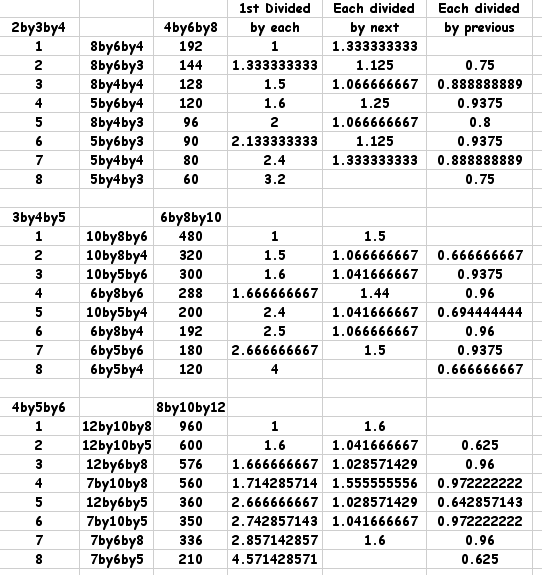#### You may also like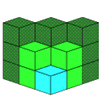If you had 36 cubes, what different cuboids could you make?### Construct-o-straws

Make a cube out of straws and have a go at this practical challenge.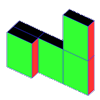### Cereal Packets

How can you put five cereal packets together to make different shapes if you must put them face-to-face?

# Cuboid-in-a-box

## Cuboid-in-a-box

We have a really good box to store things in.
It's a cuboid that's 12 cm long, 8 cm wide and 10 cm high.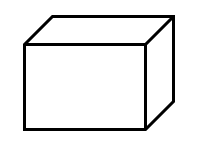We so often get asked for the biggest something or other, so for a change, here's a challenge with a difference:

What is the SMALLEST CUBOID that you can put in the box so that you cannot fit another that's the same into it?

You could use little cubes that are 1 cm wide, long and high that clip together or make a cuboid that must be a whole number of cm wide, long and high.

Let us know what you come up with - and what is more important - how you came to get a solution.

### Why do this problem?

This is a very practical challenge. You may want to let children investigate it over a series of lessons or even during an entire day. It will help them to reinforce their understanding of properties of cuboids and to be practise calculating volume. However, this is also a great opportunity for you to emphasise the power of working systematically and using visualisation to solve a problem.

### Possible approach

It will be necessary to introduce this investigation to the whole group in a practical way to make sure that they understand what the challenge is. Having a box of the correct size already made will be helpful - of course you could alter the dimensions you work with to suit some boxes you have to hand. You could begin by making a small cuboid out of cubes and asking the children to estimate how many of your cuboids might fit in the box. Try a few examples like this, using bigger cuboids each time so that it becomes harder to tell whether two will fit in or not. End with an example which is obviously too big to be fitted in the box more than once. You can then introduce the question itself.

We would recommend that children work in pairs or small groups for this activity. The quality of discussion will help to inform your assessment of the children and it would be useful to bring the whole class together at suitable intervals to talk about their ideas.

Well what I've seen often goes something like this: 1st attempt - after some initial thoughts, it needs to be a little bigger than half, so let's put a cuboid onto the base and let it be half (plus 1) high! So we get $12\;\text{cm} \times8\;\text{cm} \times6\;\text{cm}$ which has a volume of $576 \;\text{cm}^3$.

2nd attempt - a similar idea but what about a little bigger than half but up against the narrow side, so let's put a $7\;\text{cm} \times 8\;\text{cm} \times 10\;\text{cm}$ cuboid in, which has a volume of $560 \;\text{cm}^3$.

3rd attempt - well there will be a third one in this way that goes against the long side, so we'll put in a $12\;\text{cm} \times 5\; \text{cm} \times 10\;\text{cm}$ cuboid which has a volume of $600 \; \text{cm}^3$. These diagrams show these three attempts: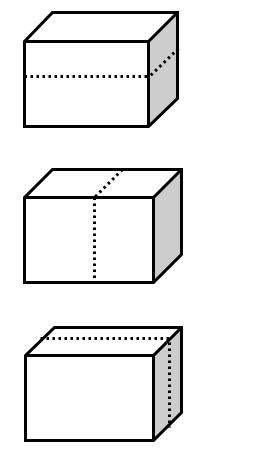4th attempt - can we combine two of these previous ideas to make something a bit bigger than a quarter of the volume of the box? We'll try a $7\;\text{cm} \times 8\;\text{cm} \times 6\;\text{cm}$ with a volume of $336 \; \text{cm}^3$ that's much better. BUT can we fit another one in?

### Possible extension

Here's a bit of extension work.
If you work on the first ideas of taking half of one of the dimensions and adding 1, and then go on to combining two dimensions and finally three there are 7 possibilities. Moving away from the original challenge we can investigate these 7 cuboids.

I've been exploring three different sizes of boxes, $4 \times6 \times 8$,$6 \times8 \times10$, and $8 \times10 \times 12$ - each being double a set of consecutive numbers.

The next stage was to look at the volumes of each of the cuboids together with the boxes they were to go into making 8 altogether.

Then I explored various divisions.

This kind of exploration developing from a practical situation can lead some of the more able pupils into unexplored areas of number patterns!